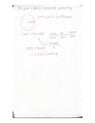# Calculating how many revolutions it takes to reach the final speed

• haven
In summary, the merry-go-round reached its final speed by rotating around its center of mass (COM) for 1.15 rotations.

#### haven

Homework Statement
I don't understand what I am doing wrong when solving this problem. The radius of the circle is 2.6 meters which means the circumference is 2pi*2.6. If the final angular velocity is 11 rpm how do I find how many revolutions it took?
Relevant Equations
1 rev = 2pi
11 rpm equals 1.15 rad/ sec not sure how this helps contribute to the question but I solved for it just in case

What's the question ?

•berkeman
Dylan exerts a constant force of 98 N on a merry-go-round 15° from the tangential direction and is able to accelerate the ride and his friends from rest to a final angular speed of 11 rpm. The merry-go-round has a mass of 850 kg, a radius of 2.6 m, and a radius of gyration about the center of 1.9 m. Corbin (67 kg) and Andrew (63 kg) sit opposite each other on the edge of the ride.

How many revolutions did it take the merry-go-round to get to its final speed?

haven said:
Homework Statement:: I don't understand what I am doing wrong when solving this problem. The radius of the circle is 2.6 meters which means the circumference is 2pi*2.6. If the final angular velocity is 11 rpm how do I find how many revolutions it took?
Relevant Equations:: 1 rev = 2pi

11 rpm equals 1.15 rad/ sec not sure how this helps contribute to the question but I solved for it just in case
Welcome to PF.As already mentioned, you did not post the full text of the problem statement. We need that in order to help you. We also need to see all of your calculations on the problem, in order to check those and provide hints for fixes/improvements.

haven said:
Dylan exerts a constant force of 98 N on a merry-go-round 15° from the tangential direction and is able to accelerate the ride and his friends from rest to a final angular speed of 11 rpm. The merry-go-round has a mass of 850 kg, a radius of 2.6 m, and a radius of gyration about the center of 1.9 m. Corbin (67 kg) and Andrew (63 kg) sit opposite each other on the edge of the ride.

How many revolutions did it take the merry-go-round to get to its final speed?
And your work on the solution?

berkeman said:
And your work on the solution?

#### Attachments

•Scanned Documents 6.jpg
16.7 KB · Views: 41
attachments

There are forces, torques, moment of inertia in this problem. The angular velocity of the Merry-go-round is not constant. You must apply Newton’s Laws.

Okay, nice work on the dimensional analysis - that may come in handy - but, I don't think the rest of it is necessary to solve the problem (I could be wrong : I haven't done it, myself). Can you see your way from where you're at, to the solution ?

If you don't mind me paraphrasing, you've been given :

- a disk, of
- a certain radius, mass, and​
- a couple extra masses at the edge of the disk ;​
and
- a force,
- applied angled tangentially,​
- until it reaches a final angular velocity.​

You're asked to find how many revolutions it takes to reach that final angular velocity.

The equations for constant angular acceleration are analogous to those for constant linear acceleration ("SUVAT").
You have an initial (angular) velocity, a final (angular) velocity, and a constant (angular) acceleration, and you want to find the (angular) distance. What SUVAT equation does that suggest?
Can you compute that acceleration?

@haven, you will need to go through various steps to answer this question. Suggestion:

1. Find the total moment of inertia.
2. Find the applied torque.
3. Using the above answers, find the angular acceleration.
4. Using the angular acceleration, find the angle (in radians) covered to reach the find speed.
5. Convert this angle to rotations.

Post your attempt for each step - one step at a time if unsure, or several steps at a time if confident.

•PeroK